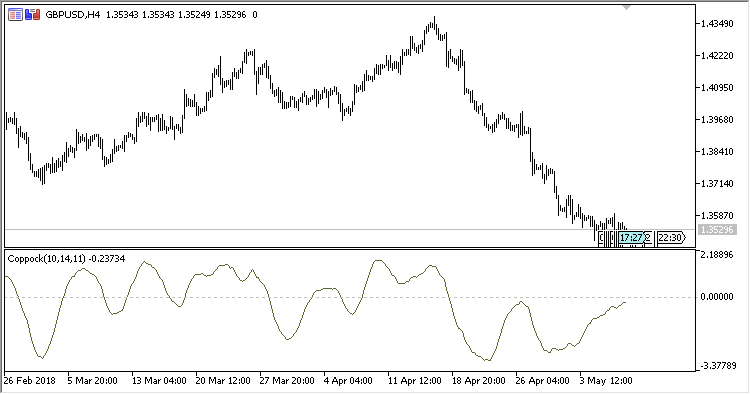# Coppock – indicator for MetaTrader 5

• A+
category：MT5 INDICATORS

The formula for calculating was proposed by Edwin Sedgwick Coppock in 1962 and published in Barron's Magazine.

The indicator is designed only for signalling about a bullish trend starting on the market. The calculation formula is not designed for bearish direction, but is sometimes is used by traders. Signal is generated when the indicator line crosses the zero level upwards.

The indicator has four input parameters:

• Period - calculation period;
• Period ROC 1 - period for calculating the first ROC (rate of price change 1);
• Period ROC 2 - period for calculating the second ROC (rate of price change 2);
• Applied price - price used for calculations.

Calculations:

`Coppock(Period) = LWMA (ROC(Period ROC 1) + ROC(Period ROC 2))`